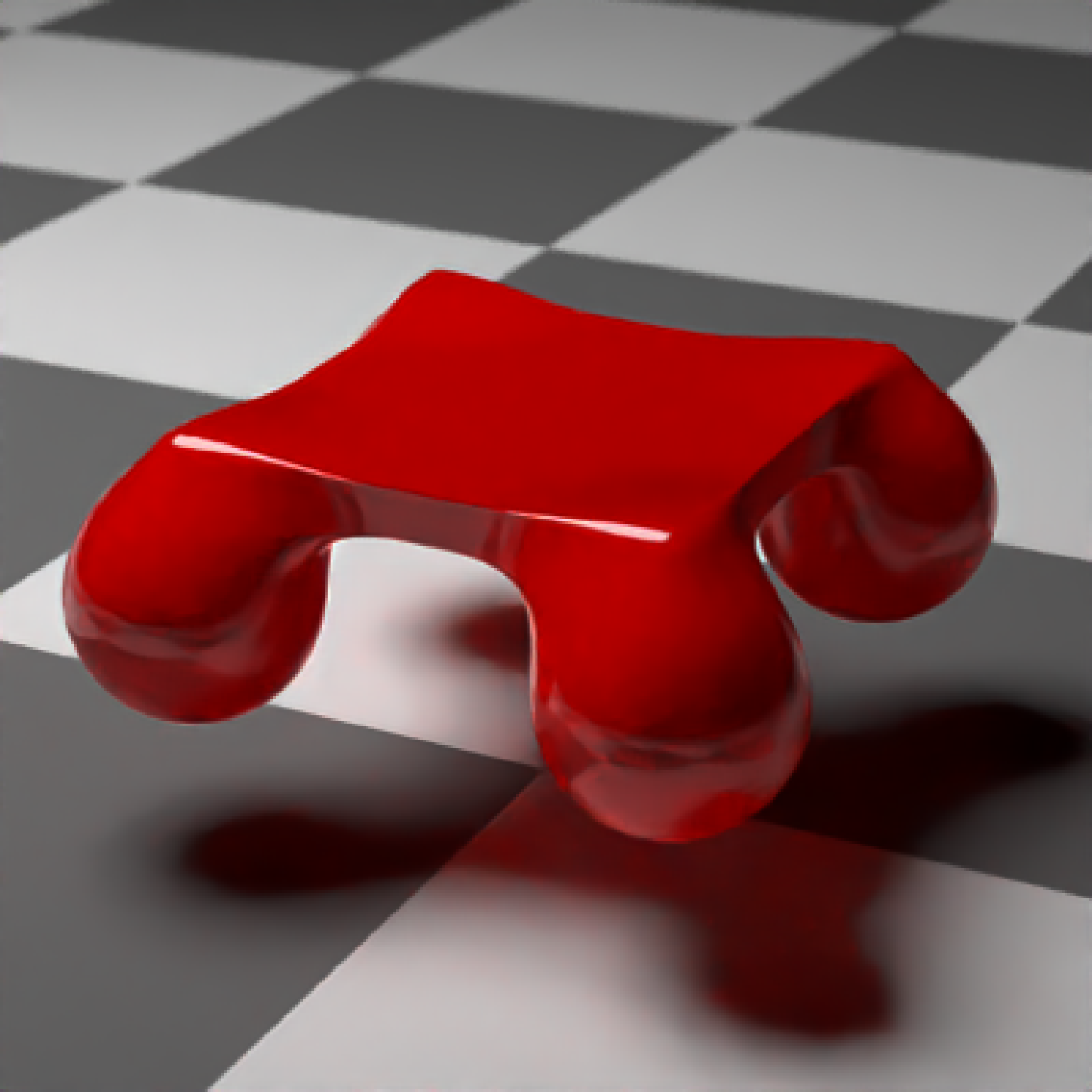CSG Group

csg_group(object_list)

## Arguments

object_list List of objects created with the csg_* functions. This will make all further operations be applied to this object as a group.

## Value

List describing the group in the scene.

## Examples

# \donttest{
#Group four spheres together and merge them with a box:
generate_ground(material=diffuse(checkercolor="grey20")) %>%
add_object(csg_object(csg_combine(
csg_group(list(csg_sphere(x=1,z=1, radius=0.5),csg_sphere(x=-1,z=1, radius=0.5),
csg_sphere(x=1,z=-1, radius=0.5),csg_sphere(x=-1,z=-1, radius=0.5))),
csg_box(y=0.5, width=c(2,0.2,2)), operation="blend"), material=glossy(color="red"))) %>%
add_object(sphere(y=10,x=-5,radius=3,material=light(intensity=10))) %>%
render_scene(clamp_value=10,lookfrom=c(5,5,10))# }